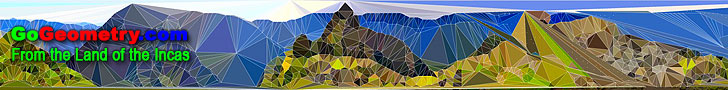# Recent additions (Page 12), Online LearningGeometry Problem 685 Isosceles Triangle, 100-40-40 Degrees, Angles, Congruence. Nagel Points Index Triangle Center Geometry Problem 684: Outer Nagel Point. Triangle, Excircles, Incircle Tangency points, Concurrent lines, Semi-perimeter, Ceva theorem. Geometry Problem 683: Nagel Point. Triangle, Excircles, Tangency points, Concurrent lines, Semi-perimeter, Ceva theorem. Geometry Problem 682: Gergonne External Point. Triangle, Excircle, Tangency points, Concurrent lines, Semiperimeter, Ceva theorem. Geometry Problem 681: Gergonne Point. Triangle, Incircle, Tangency points, Concurrent lines, Semiperimeter, Ceva theorem. Geometry Problem 680. Concentric Circles, Radii, Chords, Perpendicular, Metric Relations. Geometry Problem 679. Triangle, Circle, Median, Proportion. Geometry Problem 678. Triangle, Simson Line, Circumcircle, Tangent, Parallel, Perpendicular, Collinear Points. Geometry Problem 677. Parallelogram, Midpoint, Diagonal, Metric Relations. Geometry Problem 676. Circles, Diameter, Tangent, Chord, Metric Relations. Gergonne Points Index Triangle Center Geometry Problem 675. Intersecting Circles, Secant, 45 and 90 Degrees, Collinear Points. Geometry Problem 674. Triangle, Altitude, Angles. Geometry Problem 673. Internally tangent circles, Chord, Tangent, Metric Relations. Bulldozers: Caterpillar D8, D9, D10, D11 Exhibition. Video and News. Geometry Problem 672. Internally tangent circles, Chord, Tangent, Geometric Mean. Geometry Problem 671. Triangle, Cevian, Angles, Congruence. Geometry Problem 670. Triangle, 60 Degrees, Orthocenter, Incenter, Circumcenter, Congruence. Geometry Problems 661 - 670. Triangle, Circle, 60 Degrees, Incenter, Circumcenter, Orthocenter, Parallelogram, Metric Relations, Congruence, Similarity. Geometry Problem 669. Triangle, Circumcircle, Incenter, Midpoint, Collinear points, Mind Map. Madonna of the Goldfinch by Raphael and Golden Rectangles John the Baptist offers the goldfinch to Christ in warning of his future. Geometry Problem 668. Right triangle, Incircles, Parallel, Common Tangent, Mind Map. Geometry Problem 667. Intersecting Circles, Secant, Tangent, Metric Relations, Mind Map. Geometry Problem 666. Intersecting Circles, Secant, Tangent, Concyclic points, Mind Map. Geometry Problem 665. Intersecting Circles, Secant, Tangent, Angles, Mind Map. Geometry Problem 664. Triangle, 60 Degrees, Orthocenter, Incenter, Circumcenter, Angles, Triangle centers. Go to Page: Previous | 1 | 2 | 3 | 4 | 5 | 6 | 7 | 8 | 9 | 10 | 11 | 12 | 13 | 14 | 15 | 16 | 17 | 18 | 19 | 20 | 21 | 22 | 23 | 24 | 25 | 26 | 27 | 28 | 29 | 30 | 31 | 32 | 33 | 34 | 35 | 36 | 37 | 38 | 39 | 40 | 41 | 42 | 43 | 44 | 45 | 46 | 47 | 48 | 49 | 50 | Next

 Home | GoGeometry Education | Geometry | Search | Emai